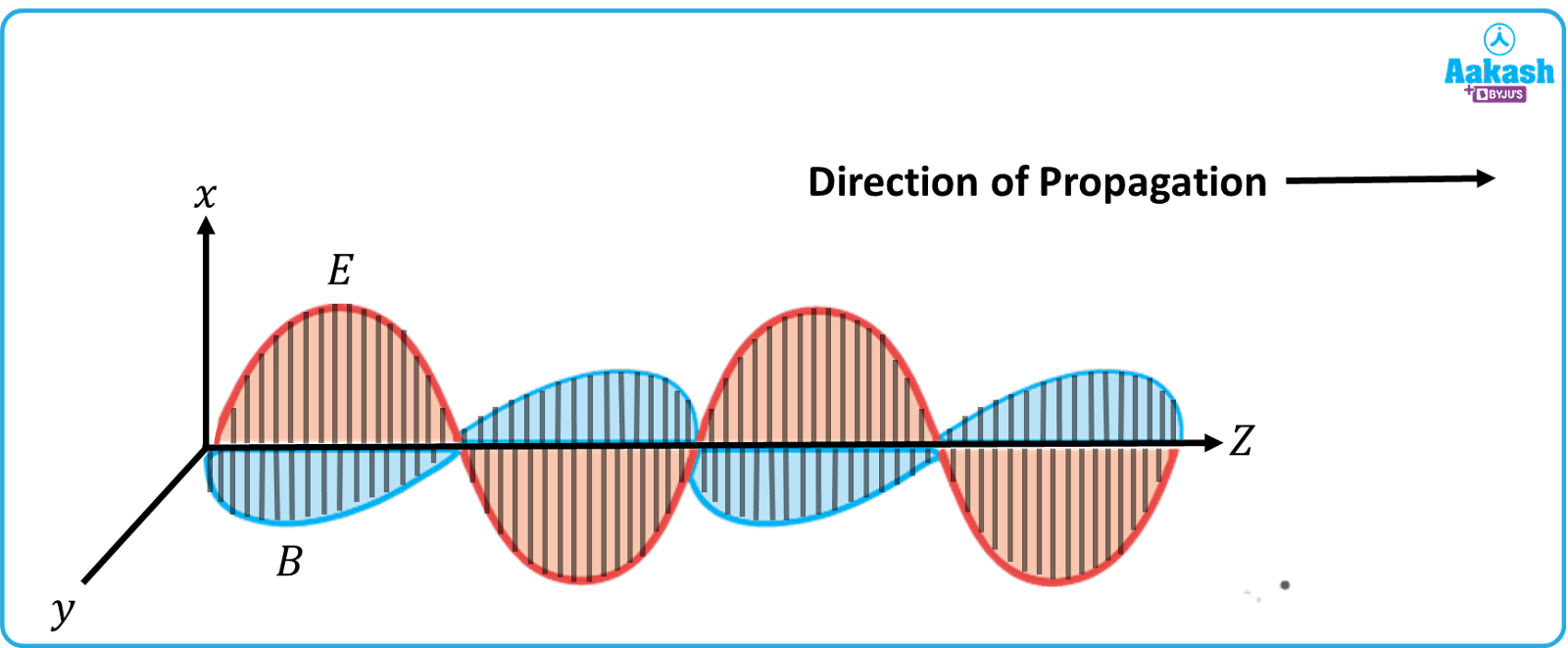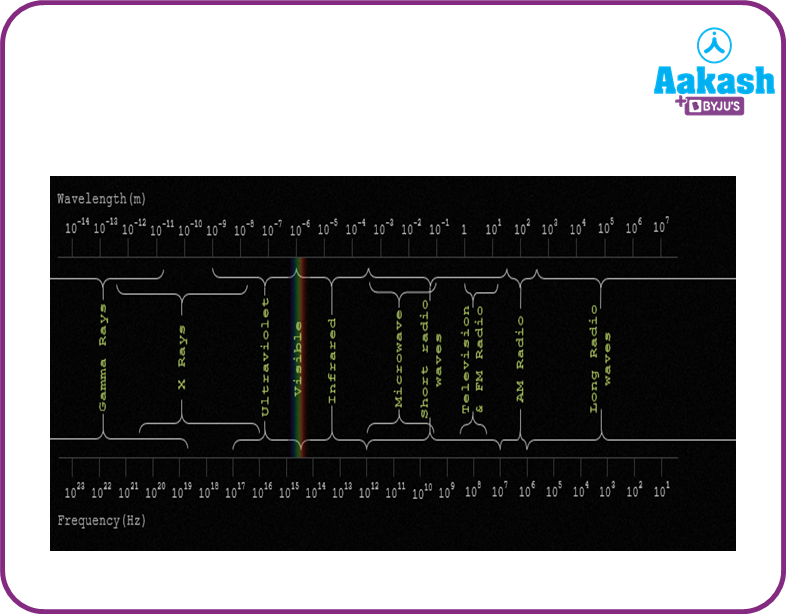• Call Now

1800-102-2727•

# Electromagnetic waves - Definition, Examples, Characteristics, Practice problems, FAQs

Starting from the smart phones we use, the sunlight we see everyday and UV lights used in laboratories to destroy microbes, electromagnetic waves are encountered everyday in life. The EM waves come under the category of non-mechanical waves – they do not need a material medium to travel through. However, the speed of the electromagnetic waves is dependent upon the medium it propagates through. EM waves were extensively studied by James Clark Maxwell. The electromagnetic spectrum refers to the entire set of radiations which include visible light, Infrared, radio waves, etc. In this article, we will explore electromagnetic waves in detail.

• Definition of EM waves
• Equation for EM waves
• Characteristics of EM waves
• Physical quantities of EM waves
• Electromagnetic spectrum
• Practice problems
• FAQs

## Definition of EM waves

The electromagnetic waves are produced due to the vibration of an electric charge. A time varying electric field gives rise to a time varying magnetic field. The vice versa is also true.

They can also be produced when high speed electrons enter into the target metal which has high atomic weight. EM waves are also produced when a radioactive nucleus de-excites.The above figure shows the propagation of EM waves through a medium. The electric field ($\stackrel{\to }{E}$) is directed along the x direction, magnetic field $\left(\stackrel{\to }{B}\right)$ is directed along y direction–hence they act perpendicular to each other, and also vary sinusoidally. The direction in which the wave would propagate is given by

$\stackrel{\to }{v}=\stackrel{\to }{E}×\stackrel{\to }{B}$

## Equation for EM waves

The equations for electric and magnetic fields are given by,

$E={E}_{x}={E}_{0}\mathrm{sin}\left(kz-\omega t\right)$
$B={B}_{y}={B}_{0}\mathrm{sin}\left(kz-\omega t\right)$

Here, k= $\frac{2\pi }{\lambda }$ is called the wave number.

$\lambda -$wavelength

$\omega =2\pi \nu$ is the angular frequency.

$\nu -$frequency in Hertz.

The speed of electromagnetic waves in vacuum or free space is given by,

$c=\frac{1}{\sqrt{{\mu }_{0}{ϵ}_{0}}}$

${ϵ}_{0}-$permittivity of free space

${\mu }_{0}-$permeability of free space

Characteristics of EM waves

1) They do not require a medium for propagation.

2) They propagate in vacuum with a speed of

3)EM waves are transverse in nature.

4) They are not deflected by electric and magnetic fields.

5)EM waves get polarized.

6)They carry energy and momentum

Physical quantities of EM waves

(i)Speed

In any other medium than air, the speed of an EM wave is given by,

$v=\frac{1}{\sqrt{{\mu ϵ}_{}}}$

$\mu ,ϵ$, indicate the permeability and permittivity of the medium respectively.

(ii)Energy

Energy density refers to the energy stored per unit volume in the wave. The energy density of the electric field is given by,

${u}_{E}=\frac{1}{2}{ϵ}_{0}{E}^{2}$

Similarly, the energy density of the magnetic field is given by

${u}_{m}=\frac{{B}^{2}}{2{\mu }_{0}}$

The total energy density is given by

${u}_{m}=\frac{{B}^{2}}{2{\mu }_{0}}+\frac{1}{2}{ϵ}_{0}{E}^{2}$

The average energy density is given by

${u}_{av}=\frac{1}{4}{ϵ}_{0}{{E}_{0}}^{2}+\frac{1}{4{\mu }_{0}}{{{B}_{0}}^{2}=\frac{1}{2}{ϵ}_{0}{{E}_{0}}^{2}=\frac{{{B}_{0}}^{2}}{2{\mu }_{0}}}^{}$

E0-peak value of electric field

B0-peak value of magnetic field

(iii)Intensity

The quantity of energy crossing per unit area per unit time through a surface, in a direction perpendicular to the surface is called intensity.

I= ${u}_{av}×c=\frac{1}{2}{ϵ}_{0}{c{E}_{0}}^{2}$

I= ; But $Power\left(P\right)=\frac{Energy}{Time}$

I= $\frac{P}{4\pi {r}^{2}}$

(iv)Momentum

The momentum of an EM wave, when it is incident on a completely absorbing surface is given by

$\mathbit{p}=\frac{\mathbit{E}\mathbit{n}\mathbit{e}\mathbit{r}\mathbit{g}\mathbit{y}}{\mathbit{S}\mathbit{p}\mathbit{e}\mathbit{e}\mathbit{d}}=\frac{\mathbit{u}}{\mathbit{c}}$

While incident on a totally reflecting surface, the momentum

$\mathbit{p}=\frac{2\mathbit{u}}{\mathbit{c}}$

(v)Magnetic and electric field

Let E0 indicate the maximum value of electric field and B0 indicate the maximum magnetic field. Then,

$c=\frac{{E}_{0}}{{B}_{0}}$

Electromagnetic spectrum

The following diagram shows the classification of EM waves. Gamma rays having least wavelength and highest frequency lie at the far end on the left. On the other hand, radio waveshaving the highest wavelength and least frequency lies on the far right end.

## Practice problems

Q1.An EM wave having frequency 40 MHz travels in vacuum in the x-direction.

(i) calculate the wavelength of the wave

(ii)At some instant of time, the electric field has a peak value of 750 NC-1 and is directed along the y-axis.

Calculate the magnitude and direction of the magnetic field?

Given,

${E}_{0}=750\frac{N}{C}.$

(i)Wavelength of the wave,

(ii)Maximum value of magnetic field,

Given,

Applying $\stackrel{\to }{v}=\stackrel{\to }{E}×\stackrel{\to }{B};$ we find that B is directed along the z-axis.

Q2. Calculate the amplitude of electric and magnetic fields in an EM wave having intensity 4.0 Wm-2.

Answer. The intensity of the EM wave is given by,

Amplitude of magnetic field,

Q3.An EM wave , traveling through vacuum is given by $E={E}_{0}\mathrm{sin}\left(kx-\omega t\right).$. Which of the following parameters is independent of wavelength?

(a)k                                                              (b)$\omega$
(c)                                                      (d)$k\omega$

The ratio

Hence, this ratio is independent of wavelength.

Q4.The rms value of the electric field of an EM wave is given by 720 NC-1. Calculate the average

total energy density of the EM wave.

(a)$6.37×{10}^{-9}\frac{J}{{m}^{3}}$                                              (b)$81.35×{10}^{-12}\frac{J}{{m}^{3}}$
(c)$3.5×{10}^{-3}\frac{J}{{m}^{3}}$                                                (d)$4.58×{10}^{-6}\frac{J}{{m}^{3}}$

Average energy density,$=\frac{1}{2}{ϵ}_{0}{{E}_{0}}^{2}=\frac{1}{2}{ϵ}_{0}\left(\sqrt{2}{{E}_{rms}\right)}^{2}={ϵ}_{0}{{E}_{rms}}^{2}$

## FAQs

Q1.Which is the most important EM wave?
Visible light is the most important EM wave; since it enables us to see things around us. Visible light is further divided into Violet, Indigo, Blue, Green, Yellow, Orange and Red. Violet has the least wavelength. On the other hand, red light has the maximum wavelength.

Q2.Why don’t EM waves not need a medium to travel?
EM waves are non mechanical waves; they do not depend on the particles of the medium to transfer momentum and energy. Instead, the wave simply propagates by the vibration of a charged particle–which produces an electric and magnetic field mutually perpendicular to each other.

Q3.How to protect against too much EM wave exposure?
To safeguard against EM radiation, one must keep electronic gadgets like cell phones and laptops away. Disable Wireless devices like WiFi, and also keep sources of EMF far away.

Q4.Which EM wave has the lowest frequency?
Radio Waves have the highest wavelength and lowest frequency. They are used in remote controlled toys, mobile phones and in communication devices like walkie-talkie.Talk to our expert
Resend OTP Timer =
By submitting up, I agree to receive all the Whatsapp communication on my registered number and Aakash terms and conditions and privacy policy